The component method is one way to add vectors.

In this example we will be adding the two vectors shown below using the component method. The vectors we will be adding are displacement vectors, but the method is the same with any other type of vectors, such as velocity, acceleration, or force vectors.

You need to know about vector components.

Be sure that you understand what we mean by an x- or y-component of a vector. This page will help you with that.

It is important to view the x- and y-components of a vector in several ways. This interactive demonstration can help you with that.

There is an vector component addition calculator below.

Click hereto go to the bottom of this page where you will find a calculator to help you check your work. The intermediate calculations in the calculator are not rounded as much as those presented below, so, the numbers will differ slightly. The numbers in the following explanation are rounded so as to not clutter the presentation.

Here is a diagram showing the method we are going to explain.

These are the vectors that we are going to add.

Some of the subscript notation used in the graphics is very slightly different than the notation used in the text. The drawing program used to generate the graphics does not easily support subscripts, so, you may see notation such as 'd1' in a picture and notation such as 'd1' in the text. Hopefully, this small difference will not cause any problems.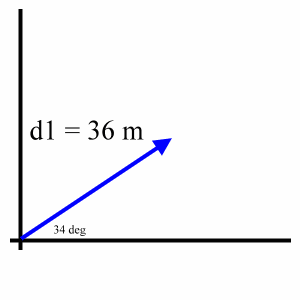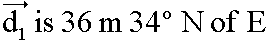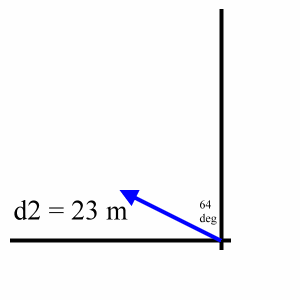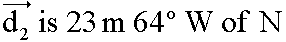The component method of addition can be summarized this way:

• Using trigonometry, find the x-component and the y-component for each vector. Refer to a diagram of each vector to correctly reason the sign, (+ or -), for each component.
• Add up both x-components, (one from each vector), to get the x-component of the total.
• Add up both y-components, (one from each vector), to get the y-component of the total.
• Add the x-component of the total to the y-component of the total, and then use the Pythagorean theorem and trigonometry to get the size and direction of the total.

Right triangle trigonometry is used to find the separate components.

Let's take this all one step at a time. First, let's visualize the x-component and the y-component of d1. Here is that diagram showing the x-component in red and the y-component in green:

The two components along with the original vector form a right triangle. Therefore, we can use right triangle trigonometry to find the lengths of the two components. That is, we can use the 'SOH-CAH-TOA' type of definitions for the sine, cosine, and tangent trigonometry functions.

Finding the first x-component.

Let's find the x-component of d1. Notice that the x-component is adjacent to the angle of 34 degrees, so, we will use the cosine function since it relates an acute angle, the adjacent side to that angle, and the hypotenuse of a right triangle: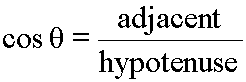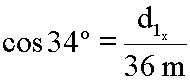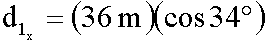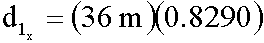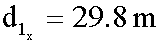Now, using trigonometry like this will not tell us the sign, (+ or -), of this component, (or any other). So, we must check the diagram for positive or negative directions. This x-component is aimed to the right, so, it is positive: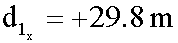(Again, remember that these calculations presented here have decimals that have been truncated. Presenting calculations to many more decimals does not help clarify methods, and, also, it violates several rules of significant digits. In other words, these calculations are approximate. The calculator below keeps many more decimal places, so, its outputs will differ slightly.)

Finding the first y-component.

Now, let's find the y-component of d1. Notice that the y-component is opposite to the angle of 34 degrees, so, we will use the sine function since it relates an acute angle, the opposite side to that angle, and the hypotenuse of a right triangle: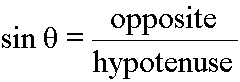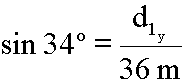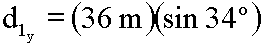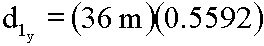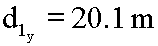Again, check the diagram for positive or negative directions. The y-component aims up, so, it is positive: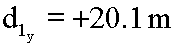The calculated values for the first set of components

Here is the diagram now showing the values for the x-component and y-component of d1:

Visualizing the components for the second vector.

Next, let's see the x-component and the y-component of d2. Here is that diagram showing the x-component in red and the y-component in green:

Calculating the second x-component

We will now find the x-component of d2. Here the x-component is opposite the angle of 64 degrees, so we will use the sine function to find it: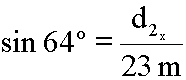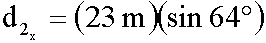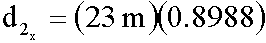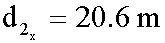Check the diagram for positive or negative directions. This x-component points to the left, so, it is negative: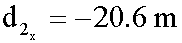Calculating the second y-component

And for our last component we will find the y-component of d2. The x-component is adjacent to the angle of 64 degrees in this diagram, so we will use the cosine function to find it: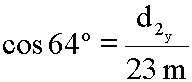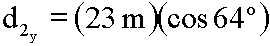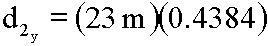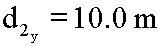Check the diagram for positive or negative directions. This y-component is aimed up, so, it is positive: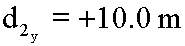Final values for the second set of components

Here is the diagram showing our newly calculated values for the components of d2:

Add all the x-components, add all the y-components, but do not add an x- to a y-component.

Now, we must add up like components to get the components for the total displacement.

To get the total x-displacement, add up all of the separate x-components: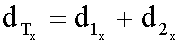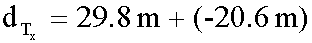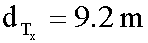To get the total y-displacement, add up all of the separate y-components: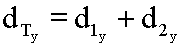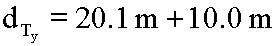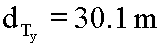So, when these two vectors, d1 and d2, are added, the total, or sum, has an x-component of 9.2 meters and a y-component of 30.1 meters.

Put together the components of the total to create the total two dimensional vector.

To get the actual 2-D total displacement, add the total x-displacement and the total y-displacement. Here is a diagram with the total x-component shown in red and the total y-component shown in green and the 2-D total shown in blue:

Use the Pythagorean theorem to get the magnitude (size) of the total 2-D displacement: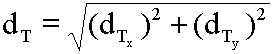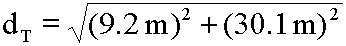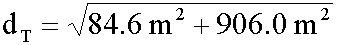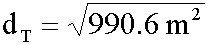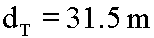Use the arctangent function to get the angle: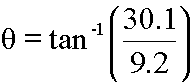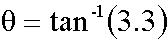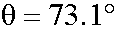Check the diagram for NSEW notation: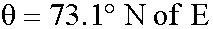Final result

Therefore, our final result for the total 2-D displacement can be stated as: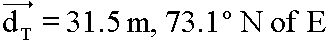Here's a diagram that shows this result:

Summary

Again, the component method of addition can be summarized this way:

• Using trigonometry, find the x-component and the y-component for each vector. Refer to a diagram of each vector to correctly reason the sign, (+ or -), for each component.
• Add up both x-components, (one from each vector), to get the x-component of the total.
• Add up both y-components, (one from each vector), to get the y-component of the total.
• Add the x-component of the total to the y-component of the total then use the Pythagorean theorem and trigonometry to get the size and direction of the total.

Make up your own vector addition problem. Create two vectors and write down their magnitudes and directions. Then, using the above described methods find their sum. Check your answer using the calculator below.

To use this calculator enter the magnitude and direction of the first and second vectors. Be sure to correctly click the radio buttons that tell the orientation for each of the direction angles. Click the 'Calculate Sum' button. The x- and y-components for each vector, the total x- and y-component for the sum, and the final magnitude and direction of the sum will be presented in a pop-up message box.

 First Vector Magnitude: Direction (degrees): N of W W of N N of E E of N S of W W of S S of E E of S

 Second Vector Magnitude: Direction (degrees): N of W W of N N of E E of N S of W W of S S of E E of S

Click this button to see the computer code for this calculator.

Here's the code that runs the above calculator.

You can change the code, if you like, and then click the following 'Reevaluate code' button. The program will then work as per your changes. Of course, your changes, especially random changes, can introduce errors, miscalculations, and browser crashes. If you need to get things back to their original condition, just reload this page using your browser's reload button.

The intention here is to conveniently show the inner workings of this program so that you understand how the calculation is done.

Click the 'Code' button again to close this section.

Custom Search